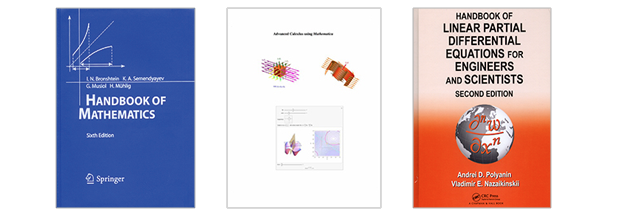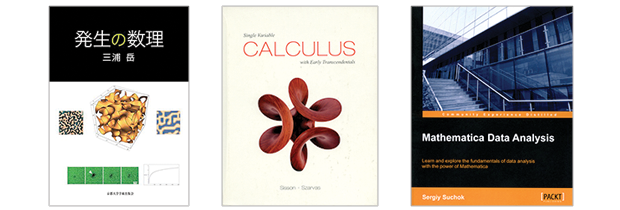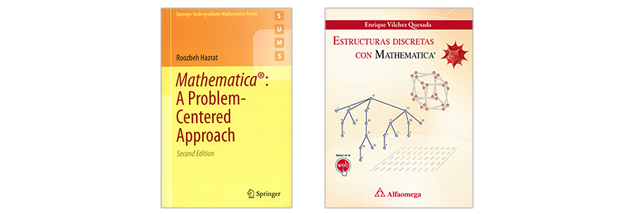Browse by Topic
Related Topics

# Newest Wolfram Technologies Books Cover Range of STEM Topics

Authors that choose to incorporate Wolfram technologies into their books are practitioners in a variety of STEM fields. Their work is an invaluable resource of information about the application of Mathematica, the Wolfram Language, and other Wolfram technologies for hobbyists, STEM professionals, and students.Handbook of Mathematics, sixth edition

This guidebook to mathematics by I. N. Bronshtein, K. A. Semendyayev, G. Musiol, and H. Muhlig contains a fundamental working knowledge of mathematics, which is needed as an everyday guide for working scientists and engineers, as well as for students. This newer edition emphasizes those fields of mathematics that have become more important for the formulation and modeling of technical and natural processes, namely numerical mathematics, probability theory and statistics, and information processing. Besides many enhancements and new paragraphs, new sections on geometric and coordinate transformations, quaternions and applications, and Lie groups and Lie algebras have also been included.

Advanced Calculus Using Mathematica: Notebook Edition

Keith Stroyan’s latest work is a complete text on calculus of several variables written in Mathematica notebooks. The eText has large, movable figures and interactive programs to illustrate things like “zooming in” to see “local linearity.” In addition to lots of traditional-style exercises, the eText also has sections on computing with Mathematica. Solutions to many exercises are in closed cells of the eText.

Handbook of Linear Partial Differential Equations for Engineers and Scientists, second edition

Including nearly 4,000 linear partial differential equations (PDEs) and a database of test problems for numerical and approximate analytical methods for solving linear PDEs and systems of coupled PDEs, Andrei D. Polyanin and Vladimir E. Nazaikinskii have created a comprehensive second edition of their handbook. The book also covers solutions to numerous problems relevant to heat and mass transfer, wave theory, hydrodynamics, aerodynamics, elasticity, acoustics, electrodynamics, diffraction theory, quantum mechanics, chemical engineering sciences, electrical engineering, and other fields.Mathematical Science of the Developmental Process (Japanese)

This book by Takashi Miura uses Mathematica to introduce and explore the developmental process, in which a simple, spherical, fertilized egg becomes a complex adult structure. The process is very difficult to understand, and the mechanism behind it has yet to be elucidated. Since no fundamental equation of this process has been established, we need prototyping processes, which means quick formulation of simple phenomenological models and verification by simulation and analysis.

Single Variable Calculus with Early Transcendentals

A comprehensive, mathematically rigorous exposition, this text from Paul Sisson and Tibor Szarvas blends precision and depth with a conversational tone to include the reader in developing the ideas and intuition of calculus. A consistent focus on historical context, theoretical discovery, and extensive exercise sets provide insight into the many applications and inherent beauty of the subject.

Mathematica Data Analysis

If you are not a programmer but you need to analyze data, Sergiy Suchok’s new book will show you how to use Mathematica to take just a few strings of intelligible code to solve huge tasks, from statistical issues to pattern recognition. If you’re a programmer, you will learn how to use the library of algorithms implemented in Mathematica in your programs, as well as how to write algorithm testing procedures. Along with intuitive queries for data processing and using functions for time series analysis, we will highlight the nuances and features of Mathematica, allowing you to build effective analysis systems.Mathematica: A Problem-Centered Approach, second edition

The second edition of Roozbeh Hazrat’s textbook introduces the vast array of features and powerful mathematical functions of Mathematica using a multitude of clearly presented examples and worked-out problems. Based on a computer algebra course taught to undergraduate students of mathematics, science, engineering, and finance, the book also includes chapters on calculus and solving equations, as well as graphics, thus covering all the basic topics in Mathematica. With its strong focus on programming and problem solving, and an emphasis on using numerical problems that do not require any particular background in mathematics, this book is also ideal for self-study and as an introduction for researchers who wish to use Mathematica as a computational tool.

Estructuras Discretas con Mathematica (Spanish)

This book by Enrique Vilchez Quesada provides a theoretical and practical overview for students studying discrete structures within the curriculum of computer engineering and computer science. The major contribution of this work, compared to other classical textbooks on this subject, consists of providing practical solutions to real-world problems in the context of computer science by creating different examples and solutions (programs, in most cases) using the renowned commercial software Mathematica.

Looking for more Wolfram technologies books? Don’t forget to visit Wolfram Books to browse by both topic and language!